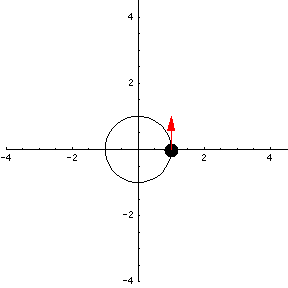# Math Insight

### Image: Parametrization of a circle 3An animation of the parametrization $\dllp(t) = (\cos t, \sin t)$ of the unit circle, with dot $\dllp(t)$ at time time and vector $\dllp'(t)$ showing velocity.

Image file: parametrization_circle_3.gif